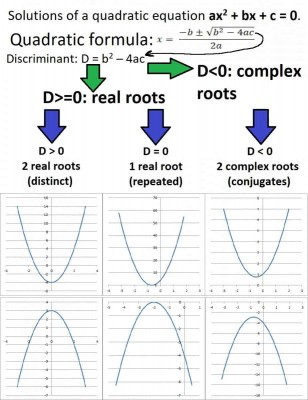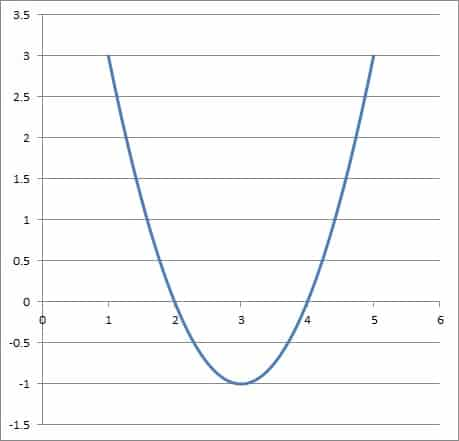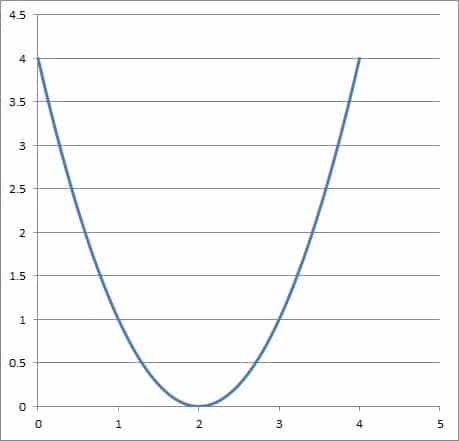# When Does A Quadratic Have Real Roots? (3 Ways To Tell)

When working with quadratic equations, the roots (solutions) can be either real or complex.  It is helpful to know what signs to look for to tell when the solutions are real.

So, when does a quadratic have real roots?  A quadratic equation has real roots when the discriminant is positive or zero (not negative).  From an algebra standpoint, this means b2 >= 4ac.  Visually, this means the graph of the quadratic (a parabola) touches the x axis at least once.

Of course, a quadratic that touches the x axis only once, at the vertex, has one repeated real root, instead of two distinct real roots.

In this article, we’ll talk about how you can tell that a quadratic has real solutions.  We’ll also look at some examples, along with how to write a quadratic equation given its real roots.

Let’s begin.

## When Does A Quadratic Have Real Roots?

There are a few ways to tell when a quadratic equation has real roots:

• Look at the discriminant – if it is positive or zero, the roots are real.
• Look at the graph – if the parabola touches the x-axis, then the roots are real.
• Look at the coefficients – there are some special cases that will tell you when there are real solutions to the quadratic (more on this later in the article!)

We’ll start with a method that uses the discriminant.

When Does A Quadratic Have No Solut...
When Does A Quadratic Have No Solution?

### Look At The Discriminant

The first way to tell if a quadratic has real roots is to look at the discriminant.  If the discriminant is positive or zero, then the quadratic equation has real roots.The discriminant is the expression b2 – 4ac under the radical in the quadratic formula. Its sign can tell us the nature of the solutions of the corresponding quadratic equation.

Remember that for the quadratic equation:

• ax2 + bx + c = 0

the discriminant is given by the expression:

• b2 – 4ac

To get a positive or zero discriminant, we need:

• b2 – 4ac >= 0

or:

• b2 >= 4ac

There are really two distinct cases when a quadratic has real roots:

• The first case is when the discriminant is positive (b2 – 4ac > 0) – this gives us two distinct real roots.
• The second case is when the discriminant is zero (b2 – 4ac = 0) – this gives us one repeated real root.

#### Discriminant Is Positive (Two Distinct Real Roots)

Here is one example of a quadratic equation with two real roots:

• 2x2 + 10x + 8 = 0

In this case, a = 2, b = 10, and c = 8.  This gives us:

• b2
• = (10)2
• = 100

and:

• 4ac
• = 4(2)(8)
• = 64

So, b2 – 4ac > 0 (since 100 – 64 = 36 > 0), and thus the discriminant is positive.  This means that the quadratic has two distinct real roots.

The two real roots are -1 and -4. You can see this by factoring the quadratic as 2(x + 1)(x + 4) = 0.

(If you wish, you can verify these solutions by using the quadratic formula with a = 2, b = 10, and c = 8).

#### Discriminant Is Equal To Zero (One Repeated Real Root)

Here is one example of a quadratic equation with one repeated real root:

• x2 + 4x + 4 = 0

In this case, a = 1, b = 4, and c = 4.  This gives us:

• b2
• = (4)2
• = 16

and

• 4ac
• = 4(1)(4)
• = 16

So, b2 – 4ac = 0 (since 16 – 16 = 0), and thus the discriminant is zero.  This means that the quadratic has one repeated real root.

The repeated real root is x = -2.  You can see this by factoring x2 + 4x + 4 as (x + 2)(x + 2).

(You can also use the quadratic formula with a = 1, b = 4, and c = 4 to verify this solution.)

### Look At The Graph

Another way to tell if a quadratic has real solutions is to look at its graph.  For any quadratic equation, the graph will be a parabola.

Remember that one of the key features of a parabola is its vertex.  The vertex of a parabola is sort of like the “mountain top” (for negative values of a) or “valley bottom” (for positive values of a).

If the parabola touches the x axis, then the quadratic has real solutions.  There are two possible cases:

• The parabola touches the x axis twice (this gives us two real roots)
• The parabola touches the x axis once, at the vertex (this gives us one repeated real root)

#### Graph of the Quadratic Touches The X Axis Twice (Two Real Roots)As you can see, the graph of the function f(x) = x2 – 6x + 8 is a parabola that touches the x axis twice, at x = 2 and x = 4. This means the quadratic equation has two distinct real roots (in this case, the discriminant is positive).

As you can see in the graph pictured above, the parabola touches the x axis in two different places. This means the quadratic equation x2 – 6x + 8 has two real roots, x = 2 and x = 4 (that is, both of the x values where the parabola and x axis intersect).

Be careful: for a quadratic equation to have two real roots, its graph must touch the x axis twice.

If the graph touches the x axis once, then the quadratic has one repeated real root (see the case below).  If the graph does not touch the x axis at all, then the quadratic has no real roots (instead, it has two distinct complex roots).

#### Graph of the Quadratic Touches The X Axis Once (One Repeated Real Root)As you can see, the graph of the function f(x) = x2 – 4x + 4 is a parabola whose vertex (valley bottom) lies on the x axis at x = 2. This means the quadratic equation has one repeated (double) real root. In this case, the discriminant is zero.

As you can see in the graph pictured above, the vertex (valley bottom) of this parabola lies on the x axis. This means the quadratic equation x2 – 4x + 4 has one real solution.

Be careful: for a quadratic equation to have one solution, its graph must touch the x axis exactly once.

If the graph touches the x axis twice, then it has two real solutions.

If the graph does not touch the x axis at all, then it has two complex solutions (and no real solutions).

### Look At The Coefficients

You can also look at the coefficients of a quadratic equation in standard form to tell if it has real roots.  Remember that the standard form of a quadratic equation has zero on one side, and terms in descending order on the other:

• ax2 + bx + c = 0

There are a few different cases to consider here.

#### When The Coefficient of x2 Is Equal To 1 (a = 1)

In this case, we look for b2 >= 4c.  If this is true, then the quadratic has real roots.

For example, the quadratic equation x2 + 4x + 3 = 0 has a = 1, b = 4, and c = 3.

This gives us:

• b2
• = 42
• = 16

and

• 4ac
• = 4*1*3
• = 12

Then b2 > 4ac (since 16 > 12), and so there are two distinct real roots for this quadratic: x = -1 and x = -3.

#### When The Coefficient of x2 Is Not Equal To 1 (a is not equal to 1)

In this case, divide the entire quadratic equation by a.  Then, you are in the first case, when the coefficient of x2 is equal to 1.

For example, take the equation 3x2 + 24x + 48 = 0.  Since a = 3, we will divide both sides by 3.

This leaves us with x2 + 8x + 16 = 0.  For this new equation, a = 1, b = 8, and c = 16.

Then we have:

• b2
• = 82
• = 64

and

• 4ac
• = 4*1*16
• = 64

This means that b2 = 4ac, so there is one repeated real root, which is x = -4.

#### When The Coefficient Of X Is Equal To 0 (b = 0)

In this case, our discriminant simplifies to -4ac.  To get real roots, the discriminant must be positive or zero.

This means -4ac >= 0.  There are two cases to consider:

• If a and c have opposite signs (one is positive and the other is negative), then the quadratic equation has real roots.
• If a and c have the same signs (both are positive or both are negative), then the quadratic equation has two complex roots.

For example, the quadratic equation x2 – 4 = 0 has two real roots.  In this case, a = 1, b = 0, and c = -4.

Since b = 0 and also a and c have opposite signs (a is positive, c is negative), we know there are real roots.  In fact, the solutions are 2 and -2.

You can verify this with the quadratic formula, or by factoring as a difference of squares: (x+ 2)(x – 2) = 0.

Remember that you can always use a calculator to help you verify the solutions to a quadratic equation.  You can also use a quadratic equation solver, such as this one from WolframAlpha.

For WolframAlpha’s calculator, remember that:

• The quadratic coefficient (x2 coefficient) means a
• The linear coefficient (x coefficient) means b
• The constant coefficient means c

## Examples of Quadratic Equations With Real Roots

Here are some examples of quadratic equations with real roots.  Look at them to see if you notice a pattern before reading further.

• x2 + x – 2 = 0 (solutions: x = 1 and x = -2)
• x2 + 2x – 3 = 0 (solutions: x = 1 and x = -3)
• x2 + 3x – 4 = 0 (solutions: x = 1 and x = -4)
• x2 + 4x – 5 = 0 (solutions: x = 1 and x = -5)
• x2 + 5x – 6 = 0 (solutions: x = 1 and x = -6)

One thing you might notice is that the x2 coefficients (a values) are equal to 1.  This simplifies the discriminant to b2 – 4c.

Another thing you might notice is that the x coefficients (b values) are all whole numbers:

• 1, 2, 3, 4, 5

You might also notice is the constant terms (c values) are negative integers:

• -2, -3, -4, -5, -6

This guarantees that the discriminant will be positive (which gives us two distinct real roots for the quadratic).

One last thing you might notice is that the solutions are 1 and c (where c is the constant term).

After we see the pattern, we can use it to create quadratic equations with real roots.  We can pick any whole number n and create a quadratic with real roots 1 and –n.

All we need to do is FOIL (x – 1)(x + n) to find our quadratic.  This would look like:

• (x + 1)(x + n) = 0
• x2 + (n-1)x – n = 0

For instance, let’s say we choose n = 10.  Then we would need to FOIL (x – 1)(x + 10), which comes out to x2 + 9x – 10.

Then the quadratic x2 + 9x – 10 = 0 has two real roots: x = 1 and x = -10.

We can also multiply a quadratic equation by any real number (except zero) to get a new equation with the same roots.  For example, if we multiply x2 + 9x – 10 = 0 by 2, we get 2x2 + 18x – 20 = 0, which also has roots of 1 and -10.

## How Do You Write A Quadratic Equation With Given Real Roots?

Now it’s time to think about working backwards.  That means taking real numbers and writing a quadratic equation with those numbers as its solutions.

This is easy to do.  Even better, there are infinitely many such equations for a given set of roots.

Let’s say we want to write a quadratic equation with two real solutions r and s.  All we need to do is FOIL (x – r)(x – s) to find the quadratic.

This would give us the quadratic equation x2 – (r+s)x + rs.

### Example: Writing A Quadratic With Given Distinct Real Roots

Let’s say we want to find a quadratic equation with real roots 4 and 7.  Then we have r = 4 and s = 7.

The equation would be a multiple of (x – r)(x – s) = 0.  Substituting r = 4 and s = 7 gives us:

• (x – r)(x – s) = 0
• (x – 4)(x – 7) = 0

Using FOIL on the left side gives us x2 – 11x + 28 = 0. We can take any multiple of this equation (for example, we could multiply both sides by 2 without changing the solutions of x = 4 and x = 7).

## Conclusion

Now you know when a quadratic equation has real roots.  You also know what to look out for in terms of the discriminant, the graph, and the coefficients.

I hope you found this article helpful.  If so, please share it with someone who can use the information.